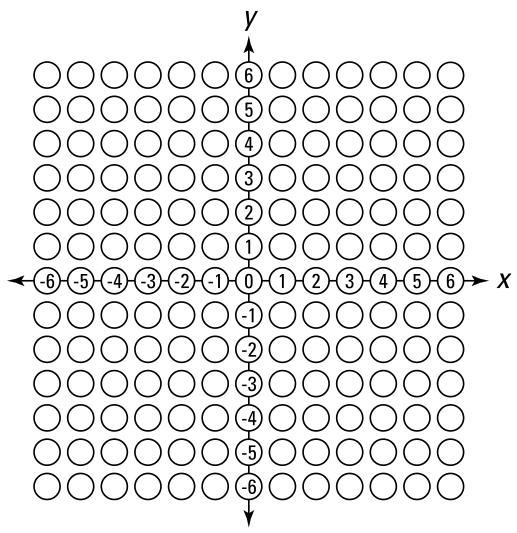##### GED Test 2022 / 2023 For Dummies with Online PracticeSome questions on the GED Math test will require you to work with points and lines on the coordinate plane. To solve these problems, you may have to sketch your results on a graph, or work with different algebraic equations. The following practice questions ask you to take both approaches.

## Practice questions

The first question refers to the following graph.

1. If the slope of AB remains the same, but it intercepts the y-axis at C (0, 4), where does it intersect the x-axis? Use the following graph to indicate the point where AB intersects the x-axis.2. The vertices of a triangle are A (–6, 4), B (–8, –6), and C (8, 7). Using graph paper, circle the two ends of the longest side.

1. The correct answer is (–6, 0). This question tests your skills in measurement and geometry. You’re asked to identify the x-intercept and the y-intercept and to draw a line with a slope of 2/3 on the graph. If you draw a line through the point on the y-axis having the same slope, it crosses the x-axis at (–6, 0). Simply count over 3 points to the left (the run), down 2 (the rise), and you’re at (–3, 2). But you’re asked for the x-intercept, so repeat this process. Go over 3 more points to the left and down 2 more, and you’re at (–6, 0).

You would fill out the answer sheet for the GED test like this:

2. The correct answer is BC. This question tests your skills in geometry by asking you to calculate the lengths of sides of a triangle when given the vertices (corners of a triangle).

The length of the line joining the points (x1, y1) and (x2, y2) is

Thus, when you substitute the three points of the triangle into this equation, you get the following lengths of AB, BC, and CA (or AC):

From these lengths, you can see that the longest side of the triangle is BC.

If you sketch this out on graph paper and locate the points of this triangle, BC is obviously the longest side.

As shown here, sketching can save you a lot of time calculating when the answer is this obvious, so try sketching first.